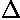## SHM p.16

### Projects for you!

The below is a list of project you should be able to complete. For some of the projects Mathematica may be of use. Here's a file containing the Mathematica I used to make these pages.

1. Hermite polynomials as orthogonal polynomials:
1. On page 3 the recursion relation for the polynomial coefficients of a Hermite polynomial are given along with an example H10 polynomial. Show that the coefficients satisfy the recursion relation. Show that H10 satisfies the differential equation we found for H.

2. Using the differential relationship on page 8 find H9 using H'10. Using "three term recursion relation" also on page 8, calculate H11 from H9 and x H10

3. On page 8 there are two "why?"s. Provide explanations.

2. On page 5 it is stated that the probability of finding a particle in an interval dx is proportional to 1/|v|. But at the classical turning point, v=0...is this a problem? Normalize the relative probability 1/|v| so that the total probability between -A and +A is one. Plot the result and compare to the plot on p.5.

3. Show that the dimensionless variables behave as if m=½ in that for example, if the dimensionless momentum p'=1, the dimensionless velocity (length/time) v'=2 and the dimensionless kinetic energy is 2. Show that in our units the period of the (1-d) motion is t'=and hencelooks like it's 2 (see page 6).

4. Fit the data of page 6 to expected classical motion and see that the quantum is like the classical.

5. Produce your own quantum moving particle following the methods of page 7.

6. Express x' and p' in terms of the raising and lowering operators of page 8. Use these results to find the following matrix elements:

<k|x'|n> <k|x'2|n>

<k|p'|n> <k|p'2|n>

<k|x'p'|n> <k|p'x'|n>

Show p'x'-x'p'=-i

Use the above results to findx' ×p' in the nth eigenstate.

7. On page 10 you saw how a set of degenerate states can be expressed differently in different coordinate systems. The five degenerate wavefunctions E'=10 could be expressed either in terms of |nrm> wavefunctions or |nxny> wavefunctions. For the three examples given, prove these results algebraically. For the 2 antisymmetric combinations (e.g., |12>-|1-2>) proceed by integration and orthogonality. Combine all the results to express each E'=10 |nrm> in terms of a sum of |nxny> wavefunctions.

8. On page 11 I claim that the minimum of the effective potential in the 2-d oscillator is r'=m½. Show this. Find the classical period of circular orbits in our dimensionless units and show the result is9. I claim on page 11 that my quantum probability blob moves uniformly in, i.e.,

We need not display similar plots for other times because the equation forshows that plot for any other time is identical to the above except rotated by 2t' radians. Thus the lump will make one complete revolution in a period ofProve this statement is true.

10. On these pages we made a "2-d" SHM problem by including a new direction (y) and a new force in the y direction: Fy=-ky. Consider instead a "2-d" SHM problem where the y direction is included, but with no force in that direction. (This is how we made ballistic motion -- a "2-d" falling problem.) Write down the energy eigenfunctions and eigenenergies. Describe the classical motion. Make a QM superposition in which a probability lump moves much like the classical motion suggests the point-particle moves.

11. On page 14 you saw WKB approximation fit into the 3-d case. Follow the same analysis for the 2-d oscillator r' equation:[Oddly enough the easiest starting point to change variables to s=ln(r') is the second equation, even though the third seems closest to WKB form.] Follow all the way through to find the WKB energies.

12. Consider a 1-d spring with an additional applied constant force (e.g., a mass-on-spring hanging in the Earth's gravitational field). Solve for the classical motion (hint: the equilibrium position just shifts, the mass will happily oscillate about the new equilibrium position just exactly as without the additional force). Following the same hint, exactly solve the Schrödinger's with the additional potential -Fx. Now treat the the additional potential as a perturbation and find the new energy levels, correct to second order.

13. The (3-d) nuclear potential is often modeled as being flatter than r2, say r4. Consider the perturbation(r4-r2) on the 3-d oscillator. When=1 we'd have our solution to the r4 potential (but low-order perturbation theory is probably not valid for such large). In any case the aim is to see how the low-energy states (say E'<=7) move as a function of. Note that since the perturbation is spherically symmetric, V' will not connect different lm states. Find the energy shifts correct to second order for the E'=7 degenerate set, and first order for E'<7. The actual order of filling in nuclei seems to be: 1s, 1p, 1d, 2s. What value ofis consistent with the data?

Note that for<0 (even slightly so) the bound states are not stable, and will "leak". Explain. What is the meaning of the perturbation theory in this case?

14. For the quarkonium states, it is believed that the potential for small r goes like -1/r and for large r goes like +r. Consider the perturbation:

V'=(r-1/r-r2)

Find the first-order shifts for the 1s, 2s, and 3s levels. Calculate the ratio of the (3s-2s) energy difference over the (2s-1s) energy difference as a function of. For the bottomonium system the ratio is (10.3553-10.0233)/(10.0233-9.460)=.59. Find the value ofthat works. For a pure oscillator this ratio is 1, what is it for the pure -1/r potential (another possible start for perturbation theory)?

15. Consider the perturbing potential V'=(x'2-y'2) in the case of the 2-d oscillator. There are 4 degenerate E'=8 states, which we may consider either in the |nxny> basis or in the |nrm> basis. Follow this problem through as we did on page 15 where we did the 3-d case.